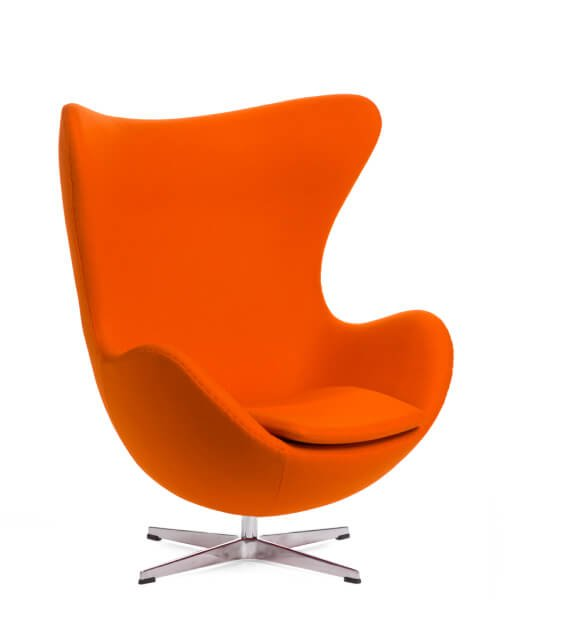•en

# What is Polygon?

Polygon is a three-dimensional geometric shape with multiple sides and angles. In 3D visualization, polygons are used to create three-dimensional models of objects. Polygons are typically made up of triangles, which are the most basic type of polygon. To create a polygon, you need to specify the vertices (points) that make up the shape. The number of sides and angles in a polygon depends on the number of vertices it has. For example, a triangle has three sides and three angles, while a square has four sides and four angles.Polygons can be either convex or concave. A convex polygon is one where all the interior angles are less than 180 degrees. A concave polygon is one where at least one of the interior angles is greater than 180 degrees.Polygons are often used in 3D visualization to create models of objects. This is because they can be used to create both simple and complex shapes. For example, a cube is made up of six square faces, which are all polygons. By creating models using polygons, we can create realistic representations of objects.Fill out the form
We will reply within 1 working day